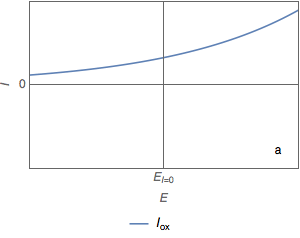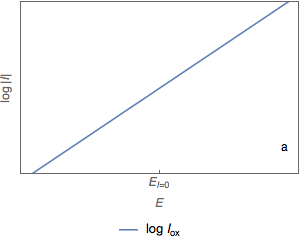Topic 5 min read# Corrosion basics: determination of the corrosion current and potential

Latest updated: June 2, 2023

Introduction

During the corrosion of a metal, the metal oxidizes according to a positive current $I_\text{ox}$ which follows an exponential law with respect to the potential $E$ (Fig. 1a).

The negative reduction current, $I_\text{red}$, of the oxidant responsible for the corrosion of the metal, oxygen or proton for example, also follows an exponential law, in the absence of limitation by the transfer of oxidant. (Fig. 1b).

The two currents $I_\text{ox}$ and $I_\text{red}$ are not measurable, only their sum $I=I_\text{ox}+ I_\text{red}$ is measurable (Fig. 1c).

The total current $I$ is zero at the corrosion potential $E_\text{corr}=E_\text{I=0}$ (Fig. 1d).

At the corrosion potential, the current $I_\text{ox}$ and the absolute value of $I_\text{red}$ are equal to the corrosion current $I_\text{corr}$ (Fig. 1e).

The value of $I_\text{corr}$ cannot be determined simply by using the $I\;vs.\;E$ curve (Fig. 1f).Figure 1: Current vs potential curves for a metal dissolved in an oxidant, partial and total currents.

Stern/Wagner-Traud method

Using a semi-logarithmic representation, where the decimal logarithm of the absolute value of the current, $\log | I|$, is plotted against the potential $E$, we have a simple method of determining the corrosion current $I_\text{corr}$.

In this representation, the graphs of the two current $I_\text{ox}$ (Fig. 2a) and $I_\text{red}$ (Fig. 2b) are straight lines.

The semi-logarithmic plot of the total current (Fig. 2c) shows two asymptotes (Fig. 2d). The abscissa of the intersection point of the two straight lines is equal to the corrosion potential $E_\text{corr}$ (Fig. 2e) and the ordinate of the intersection point is a measure of $\log I_\text{corr}$ (Fig. 2f)Figure 2: Semi-logarithmic plot of the current vs potential curves.

Ultimately, the corrosion current is used to provide the corrosion rate of an uniformely corroding sample.

The corrosion rate (CR) is calculated according to the following equation:

$\text{CR}=\frac{I_\text{corr}\text{K EW}}{dA}$

with

$\text{CR}$ in mmpy (millimeter per year) or mpy (milliinch or mil per year).

$I_\text{corr}$ the corrosion current in A.

$\text{K}$ a constant that defines the units of the corrosion rate.

It is equal to 3272 to have $CR$ in mmpy and 1.288 x 105 to have $CR$ in mpy.

$\text{EW}$, the equivalent weight in g/equivalent.

It is defined as the molar mass of the oxidized metal divided by the number of electrons involved in the dissolution reaction.

$d$ is the density of the metallic sample in g/cm3.

$A$ is the sample exposed area in cm2

For further information please see Application note number 10: Tafel plot LPR – Corrosion

corrosion current Stern Wagner-Traud Corrosion rate

Work smarter. Not harder.

Tech-tips, theory, latest functionality, new products & more.

No thanks!